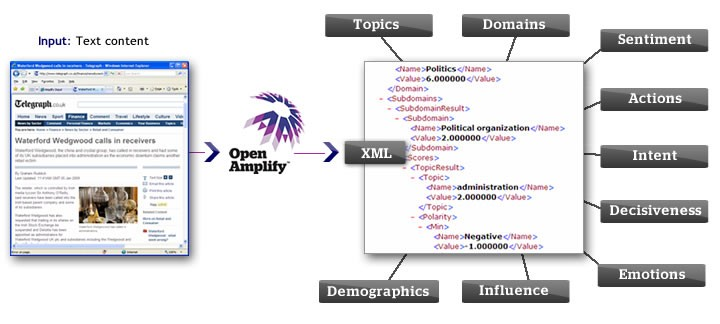# Natural Language - Document (Cosine) Similarity

Cosine similarity applied to document similarity.

## Implementation

Each document becomes a vector in some high dimensional space. To compare two documents we compute the cosine of the angle between their two document vectors.

The dot product and norm computations are simple functions of the bag-of-words document representations.

The geometric interpretation is more intuitive. When the angle between two document vectors is small, they are pointing roughly the same direction because they share many tokens in common.

• If the angle is small (they share many words in common), the cosine is large.
• If the angle is large (and they have few words in common), the cosine is small.

Discover MoreData Mining - Cosine Similarity (Measure of Angle)

The cosine similarity is a measure of the angle between two vectors, normalized by magnitude. You just divide the dot product by the magnitude of the two vectors. By taking the and definition of...NLP - Term-document Matrix

A term-document matrix is an important representation for text analytics. Each row of the matrix is a document vector, with one column for every term in the entire corpus. Naturally, some documents...